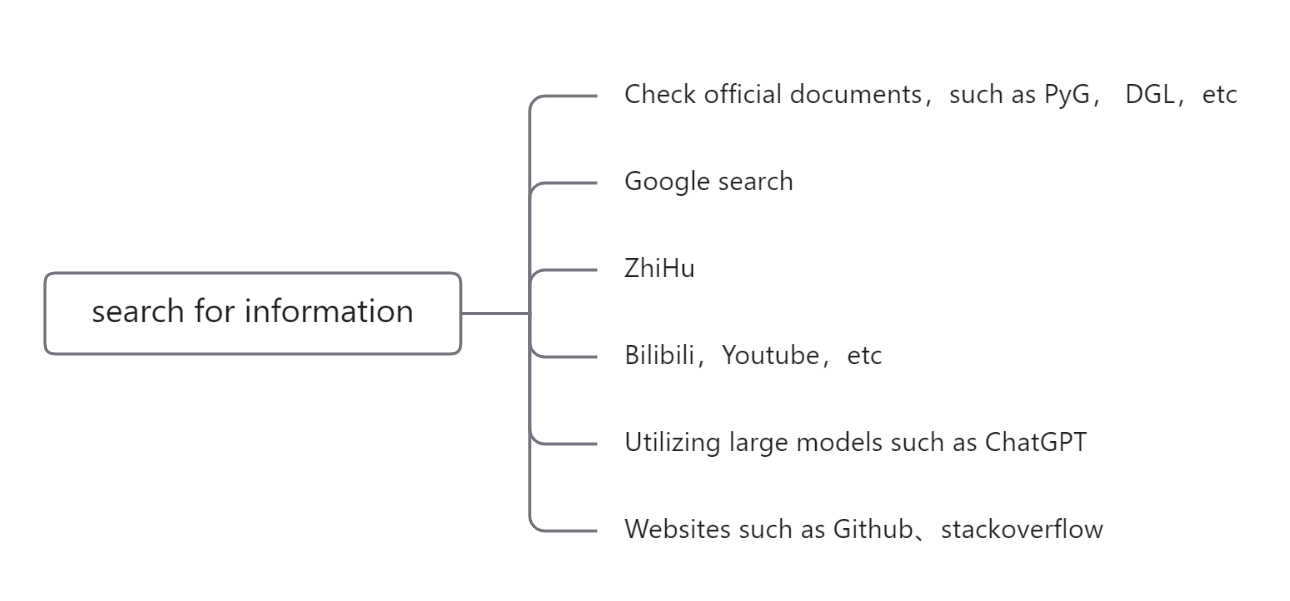## Partner Program

### Goal: Integrated graph learning algorithm based on a domestic graph learning platform

• Cultivate graph learning talents
• Promote the work of scholars
• Facilitate graph learning applications

### Learning Content

• Learn basic Python programming and master practical skills;

• Learn basic machine learning and deep learning concepts, and get familiar with deep learning frameworks such as PyTorch;

• Learn the basic theory of the graph neural network, understand the graph neural network frameworks like PyG, DGL, or GammaGL;

• Implement a graph neural network model on OpenHGNN and GammaGL using related tools.

• Interested students can go to our homepage to learn about more graph learning works.### Learning Route

#### 🐣 The First month

• The first week:

• Choose an IDEA that is easy to use, recommend Pycharm, VSCode, etc.

Master Python basic project management, project debugging, etc.

• Python3 Tutorial | Rookie Tutorial

Focus on learning the first part of the Python3 tutorial in the catalog, and those who are interested in the second part of the Python3 advanced tutorial can learn about it.

The most important content in the first part: string, list, dict, tuple, conditional control, loop statement, module, object-oriented.

Understand why "everything in Python is an object".

• Gradually learn how to bypass the wall (necessary to connect to platforms such as github).

• Complete a Python Basic exercise.

If you need related videos, you can search directly on the video website such as YouTube, .etc.

• The second week:

• Learn how to use conda to manage the Python environment.

You only need to master the simplest environment creation, the management of installing packages, and the installing channel.

• Learn to use Juypter Notebook to facilitate code writing.

• Learn commonly used libraries for data analysis: numpy, pandas, matplotlib.

It can be learned in combination with Zhihu topics.

• Complete a Data processing exercise.

• The third week:

• Combined with the above courses, you can learn and master the concepts of machine learning and deep learning such as gradient descent, backpropagation, and deep learning fast. You just need to understand and get familiar with related concepts at this stage.

• Learn the sklearn library and its related usage.

• Complete a Linear regression exercise.

• The fourth week:

• The video is only for recommendation. It is strongly recommended to follow the Tutorials and Docs of the PyTorch official website to learn. It is necessary to get familiar with the concept of tensor in PyTorch, use PyTorch to build a neural network, and use PyTorch to build a training process.

• Complete a PyTorch exercise.

#### 🐤 The Second month

• The first week

• Learn the basic concepts and the theory of graph neural networks.

• Learn about how PyG or DGL constructs graph data and constructs graph neural networks, and optionally install the above libraries.

• Learn the construction and characteristics of graph data: DGL: Graph & PyG: GET STARTED.

• Learn the core mechanism of graph neural networks-message passing: DGL: Message Passing | PyG: TUTORIALS.

Follow the examples, start writing code, and pay attention to identify the library differences between DGL and PyG (Graph-centric vs Tensor-centric).

• Complete an Algorithm library Combat.

• The second week

• How does the formula mention in GraphConv correspond to the message passing paradigm in the document (Indicate which part of the formula each symbol in the message passing paradigm corresponds to).
• For GATConv and SAGEConv, please make the same corresponding analysis.
• Which line should the formula concat mentioned in SAGEConv be in the source code, and analyze the similarities and differences between the two.
• The third week

• Reference video: Graph neural network from entry to mastery Watch some chapters selectively to learn more about the entire pipeline of graph neural networks.
• You can also refer to Chapters 3-5 of the official documentation of DGL.
• Understand GNN downstream tasks, such as node classification, link prediction, and graph classification, etc.
• Implement a working demo on PyG and DGL respectively.
• The fourth week

• Understand the two algorithm libraries GammaGL, OpenHGNN.

• GammaGL is a multi-backend library for Graph Neural Networks, which supports TensorFlow, PyTorch, PaddlePaddle and MindSpore. Users can run the same code on different deep learning backends and use tensor-centric programming styles like Pytorch Geometric (PyG). Github, Openi, Paper.
• OpenHGNN is an open source heterogeneous graph neural network toolkit based on Deep Graph Library (DGL) and PyTorch, which integrates SOTA models for heterogeneous graphs. OpenHGNN provides easy-to-use and extensible APIs, helping researchers and engineers to conduct research and applications on heterogeneous graphs fast. Github, Openi, Paper.
• Start reading classic papers step by step, such as GCN, GAT, HAN, etc.

#### The third month to the fifth month

• Understand the back-end framework used by each algorithm library.

• Implement a minimal model using the algorithm library.

• Assignment papers:

• Read the paper to understand the general content of the paper (abstract, background, problems to be solved, and general ideas, etc.);
• Compare the source code of the paper, and match the formula of the paper with the content of the code;
• Prepare the reporting slides and report the paper, generally no more than half an hour.
• Led by the responsible students, realize the model and achieve the performance of the paper.

• Reproduce the model on the specified platform according to the paper and the source code;
• Run the experiment, you should make your model performance meets the performance of the paper;
• Write the model documents.
• Find out which part of the algorithm libraries are unreasonable. We welcome your valuable suggestion.

If you are interested in this program, please email yaoqiliu@bupt.edu.cn.